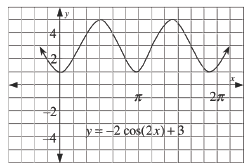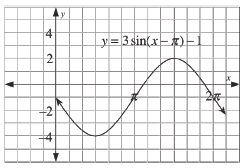### Home > PC > Chapter 4 > Lesson 4.2.3 > Problem4-105

4-105.

Describe the transformations and sketch the graphs of the following trigonometric functions.

1. $y =-2\operatorname{cos}(2x) + 3$

1. $y = 3\operatorname{sin}(x-π)-1$

Describe the amplitude, horizontal/vertical shift,
period, and orientation of the graph.

Describe the amplitude and horizontal/vertical shift of the graph.